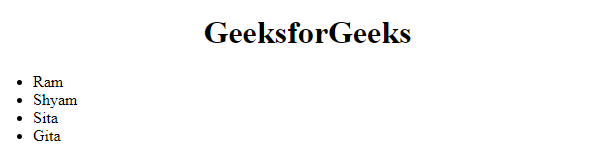Related Articles

# How to create HTML list from JavaScript array ?

• Last Updated : 10 Mar, 2021

In this article, we will be creating an HTML list from a JavaScript array. This is needed sometimes when we fetch JSON from any source and displaying the data into the frontend and in many other cases also.

Problem statement: Display the array [‘Ram’, ‘Shyam’, ‘Sita’, ‘Gita’ ] into an HTML list.

Hey geek! The constant emerging technologies in the world of web development always keeps the excitement for this subject through the roof. But before you tackle the big projects, we suggest you start by learning the basics. Kickstart your web development journey by learning JS concepts with our JavaScript Course. Now at it's lowest price ever!

To achieve this, we will follow the steps below.

• Step 1: Create the HTML skeleton.

## HTML

 ```<``html``>``  ``<``head``> ``  ``<``body``>``    ``<``center``><``h1``>GeeksforGeeks``    ``<``ul` `id``=``"myList"``>``  `````
• Step 2: Create a variable named list and get the element whose id is “myList”.

## Javascript

 `let list = document.getElementById(``"myList"``);`
• Step 3: Now iterate all the array items using JavaScript forEach and at each iteration, create a li element and put the innerText value the same as the current item, and append the li at the list.

## Javascript

 `let data = [``'Ram'``, ``'Shyam'``, ``'Sita'``, ``'Gita'` `];`` ` `let list = document.getElementById(``"myList"``);`` ` `data.forEach((item)=>{``  ``let li = document.createElement(``"li"``);``  ``li.innerText = item;``  ``list.appendChild(li);``})`

Complete code:

## HTML

 ```<``html``>``  ``<``head``> ``  ``<``body``>``    ``<``center``><``h1``>GeeksforGeeks``    ``<``ul` `id``=``"myList"``>``    ``<``script``>``      ``let data = ["Ram", "Shyam", "Sita", "Gita"];`` ` `      ``let list = document.getElementById("myList");`` ` `      ``data.forEach((item) => {``        ``let li = document.createElement("li");``        ``li.innerText = item;``        ``list.appendChild(li);``      ``});``    ````  `````

Output:My Personal Notes arrow_drop_up